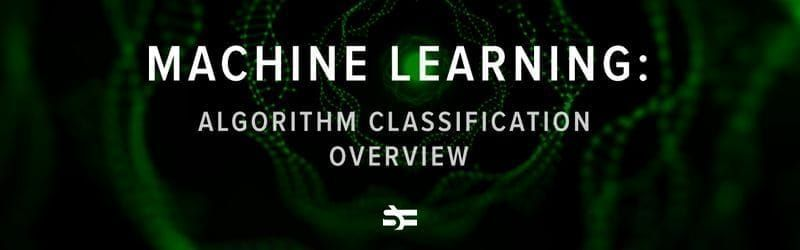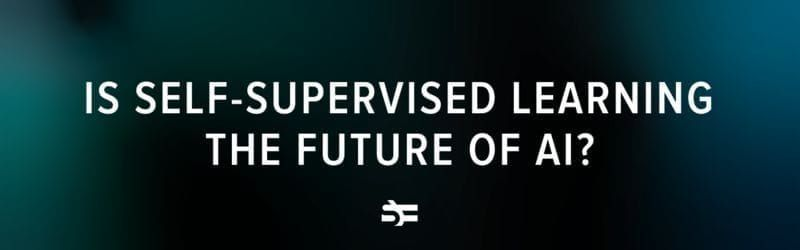# Regression Analysis Overview: The Hows and The Whys

Machine learning experts have borrowed the methods of regression analysis from math because they allow making predictions with as little as just one known variable as well as multiple variables. They are useful for financial analysis, weather forecasting, medical diagnosis, and many other fields.

## What is regression in statistics?

Regression analysis determines the relationship between one dependent variable and a set of independent variables. This sounds a bit complicated, so let’s look at an example.

Imagine that you run your own restaurant. You have a waiter who receives tips. The size of those tips usually correlates with the total sum for the meal. The bigger they are, the more expensive the meal was.

You have a list of order numbers and tips received. If you tried to reconstruct how large each meal was with just the tip data (a dependent variable), this would be an example of a simple linear regression analysis.

(This example was borrowed from the magnificent video by Brandon Foltz.)

A similar case would be trying to predict how much the apartment will cost based just on its size. While this estimation is not perfect, a larger apartment will usually cost more than a smaller one.

To be honest, simple linear regression is not the only type of regression in machine learning and not even the most practical one. However, it is the easiest to understand.

## Representation of the regression model

The representation of a linear regression model is a linear equation.

$Y = a + bX$

In this equation, $Y$ is the value that we are trying to predict. $X$ is the independent input value. Regarding parameters: $b$ represents the coefficient that every input value is multiplied with, while $a$ (the intercept coefficient) is a coefficient that we add in the end. Changing $b$ impacts the slope of the line, while changing a lets one move the line up and down the $Y$ axis.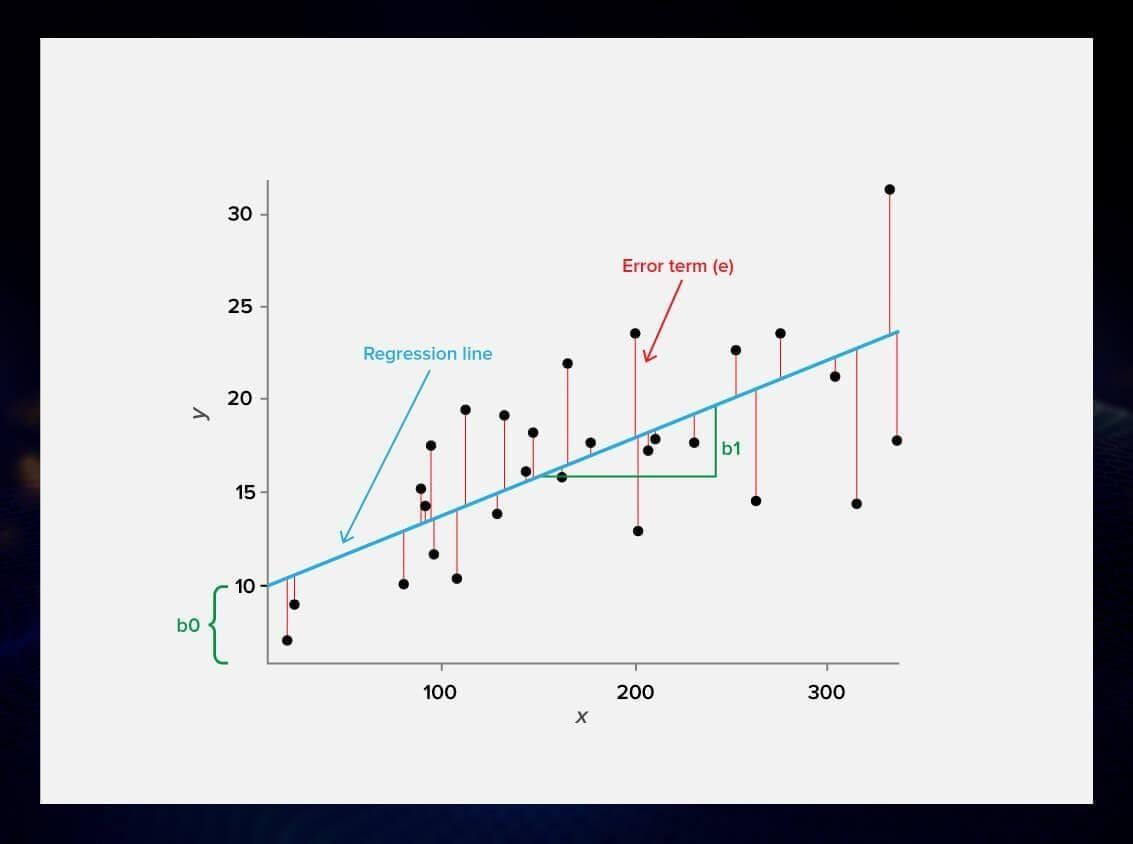## Types of regression for ML

Let’s now look at the common types of linear regression analysis that are used in machine learning. There are four basic techniques that we are going to have a look at here. Other regression models can also be found, but they are not so commonly used.

### Simple linear regression

Simple linear regression uses one independent variable to explain or predict the outcome.

For example, you have a table with the sample data concerning the temperature of cables and their durability. Now, you can do simple linear regression to create a model that can predict the durability of a cable based on its temperature.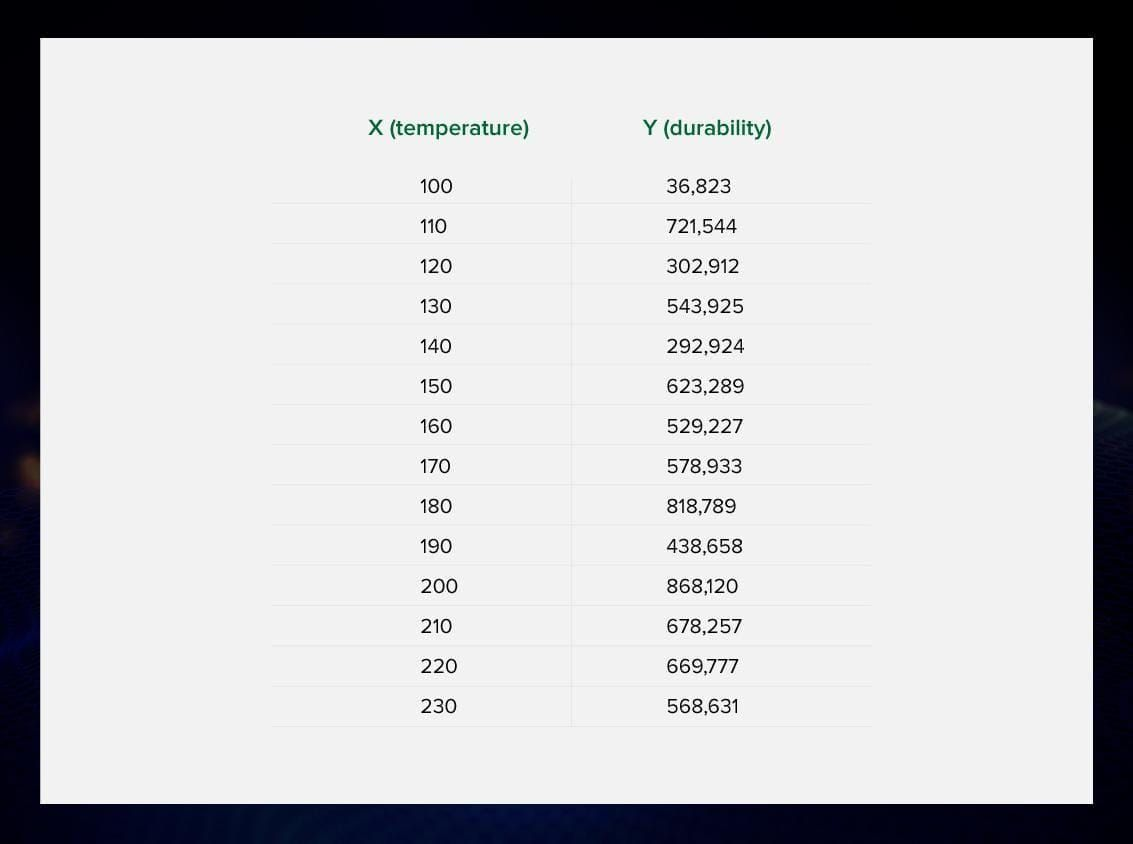The predictions you make with simple regression will usually be rather inaccurate. A cable’s durability depends on many other things than just the temperature: wear, weight of carriage, humidity, and other factors. That is why simple linear regression is not usually used to solve real-life tasks.

### Multiple linear regression for machine learning

Unlike simple linear regression, multiple linear regression uses several explanatory variables to predict the dependent outcome of a response variable.

A multiple linear regression model looks like this:

$Y = a + b_1X_1 + b_2X_2 + b_3X_3 + ... + b_tX_t$

Here, $Y$ is the variable that you are trying to predict, $X$’s are the variables that you are using to predict $Y$, $a$ is the intercept, and $b$’s are the regression coefficients – they show how much a change in certain $X$ predicts a change in $Y$, everything else being equal.

In real life, multiple regression can be used by ML-powered algorithms to predict the price of stocks based on fluctuations in similar stocks.

However, it would be erroneous to say that the more variables you have, the more accurate your ML prediction is.

#### Problems with multiple linear regression

Two possible problems arise with the use of multiple regression: overfitting and multicollinearity.

Overfitting means that the model you build with multiple regression becomes too narrow and does not generalize well. It works okay on the training set of your machine learning model but does not function properly on the items not mentioned before.

Multicollinearity describes the situation when there is correlation between not only the independent variables and the dependent variable but also between the independent variables themselves. We don’t want this to happen because it leads to misleading results for the model.

To conduct this type of analysis properly, you need to carefully prepare your data. We are going to talk about that later in this post.

### Ordinary least squares

Another method of linear regression is ordinary least squares. This procedure helps you find the optimal line for a set of data points by minimizing the sum of the residuals.

Every data point represents the relationship between an independent variable and a dependent variable (that we are trying to predict).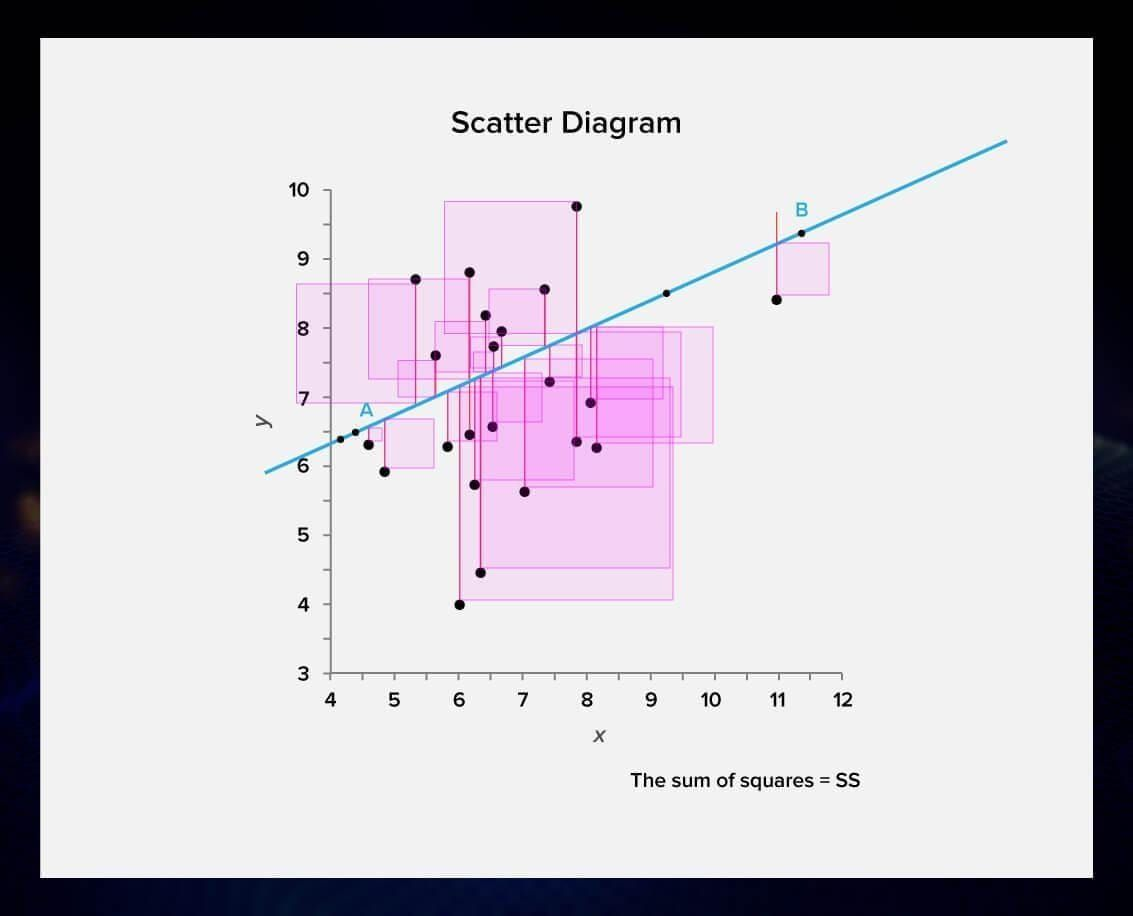To represent the regression visually, you start by plotting the data points and then draw a line that has the smallest sum of squared distances (residuals) between the line and the data points. In ordinary least squares, this is usually done by finding a local minimum through partial derivatives.

Gradient descent is used for the optimization and fine-tuning of models.

Gradient descent is the process of finding something close to the local minimum of a function by repeatedly changing the parameters in the direction where the function gives a smaller result.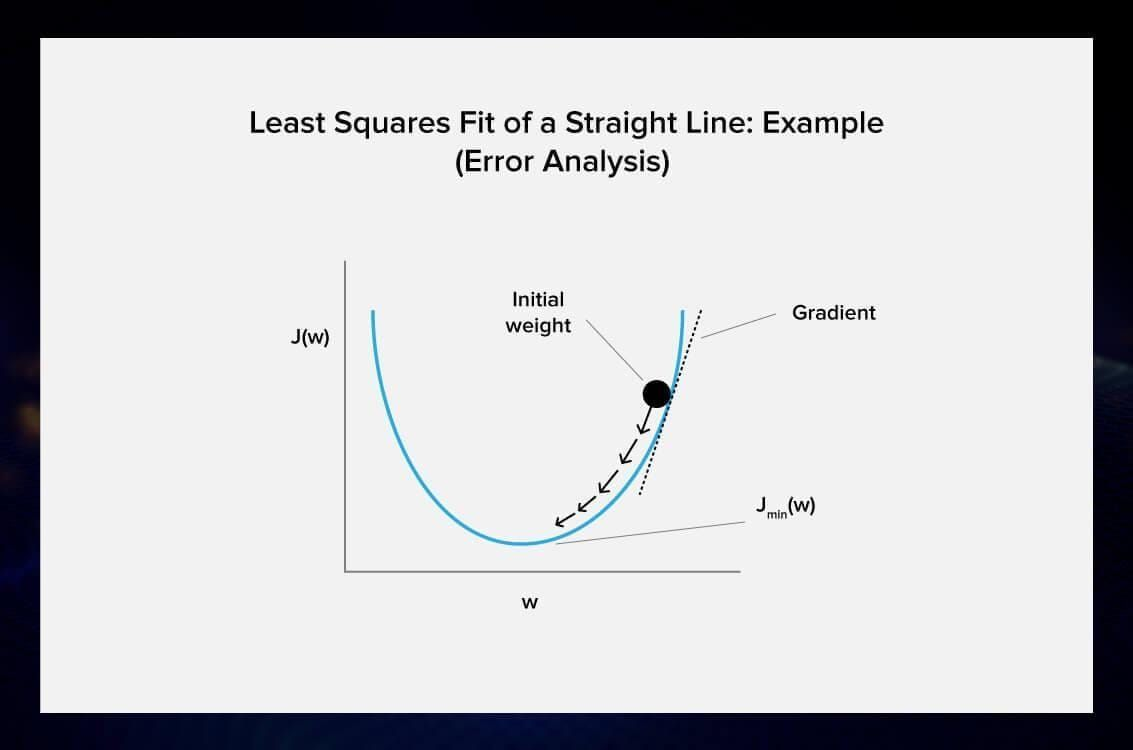In the context of linear regression, we can use it to iteratively find the line with the smallest sum of squared residuals without calculating the optimal values for our coefficients.

We start with random values for each parameter of the model and calculate the sum of squared errors. Then, we iteratively update the parameters so that the sum of squared differences is smaller than with the original parameters. We do this until the sum doesn’t decrease anymore. At this moment, the GD has converged, and the parameters we have should provide us with a local minimum.

When you apply this technique, you need to choose a learning rate that determines the size of the improvement step to take on each iteration of the procedure. The process is repeated until a minimum sum squared error is achieved or no further improvement is possible.

A learning rate is an important concept when we talk about gradient descent. It describes the size of the required step. When the learning rate is high, you can discover more information with each step, but risk impacting the accuracy. In the case of a large enough step, the GD algorithm can not converge at all. A low learning rate is more accurate but we’re recalculating the values so frequently that it becomes inefficient. Gradient descent takes a lot of time, so the increased accuracy is usually not worth it.

In practice, gradient descent is useful when you have a lot of variables or data points, since calculating the answer might be expensive. In most situations, it will result in a line comparable to one drawn by OLS.

### Regularization

This linear regression technique tries to reduce the complexity of the model by adding restrictions or pre-assumptions that help to avoid overfitting.

These regularization methods help when there is multicollinearity between your independent variables and using the ordinary least squares method causes overfitting:

• Lasso Regression: Ordinary least squares is changed to also minimize the absolute sum of the coefficients (called L1 regularization). Frequently, in the case of overfitting, we get very large coefficients. We could avoid it by minimizing not only the sum of the errors but also some function of the coefficients.
• Ridge Regression: Ordinary least squares is changed to also minimize the squared absolute sum of the coefficients (called L2 regularization).

## Data preparation and making predictions with regression

Now let us see step by step how you approach a regression problem in ML.

### 1. Generate a list of potential variables

Analyze your problem and come up with potential independent variables that will help you to predict the dependent variable. For example, you can use regression to predict the impact of the product price and the marketing budget on sales.

### 2. Collect data on the variables

Now it is time to collect historical data samples. Every company keeps track of sales, marketing budget, and prices of all the products they make. For our regression model, we need a dataset that looks like this:

 # Price in 1000$(x1) Marketing budget in 1000$(x2) Number of sales in 1000(y) 1 0.2 5 20 2 0.089 25 50 3 0.3 3 10 4 0.5 5.5 20 5 0.103 2 1 6 0.044 4 20 7 0.289 1.5 5 8 0.170 2 6 9 0.056 30 60 10 0.017 15 40

### 3. Check the relationship between each independent variable and the dependent variable using scatter plots and correlations

Placing the data points on a scatter plot is an intuitive way to see whether there is a linear relationship between the variables. I used the linear regression calculator on Alcula.com but you can use any tool you like.

Let us start with the relationship between the price and the number of sales.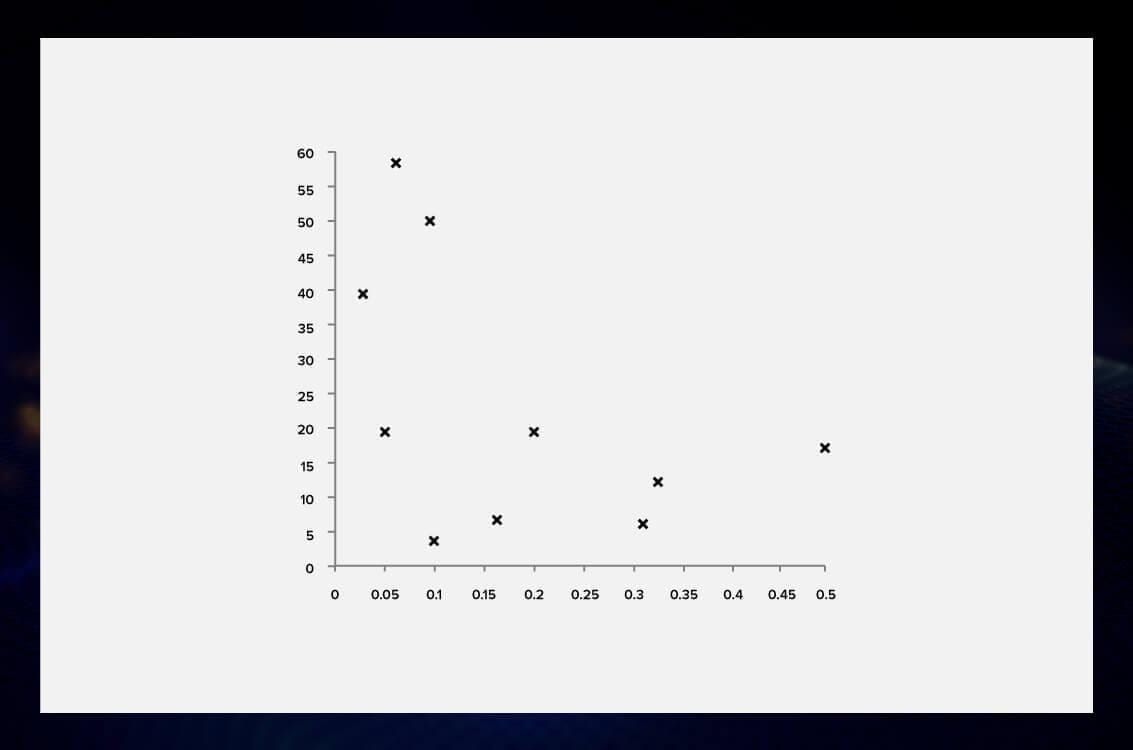We can fit a line to the observed data.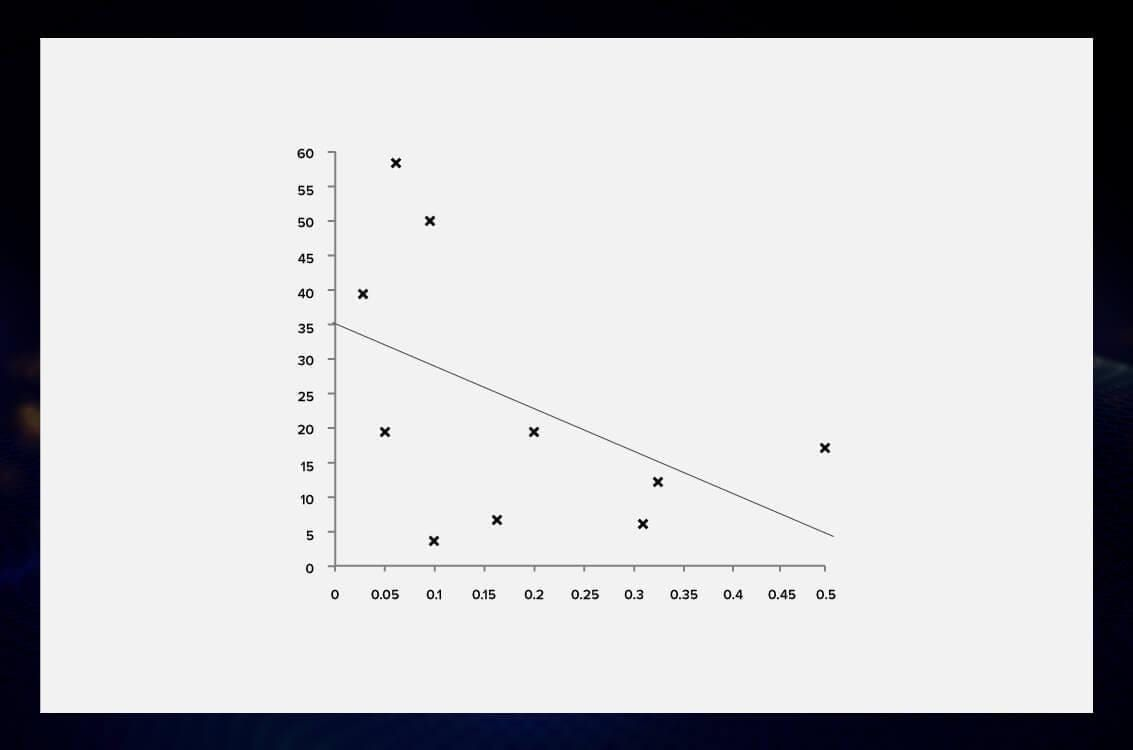Now we need to check the correlation between the variables. For that, I used an online calculator.

The correlation equals -0.441. This is called a negative correlation: one variable increases when the other one decreases. The higher the price, the lower the number of sales.

However, we also want to check the relationship between the money we invested in marketing and the number of sales.

Here is how our data points look on a scatter plot.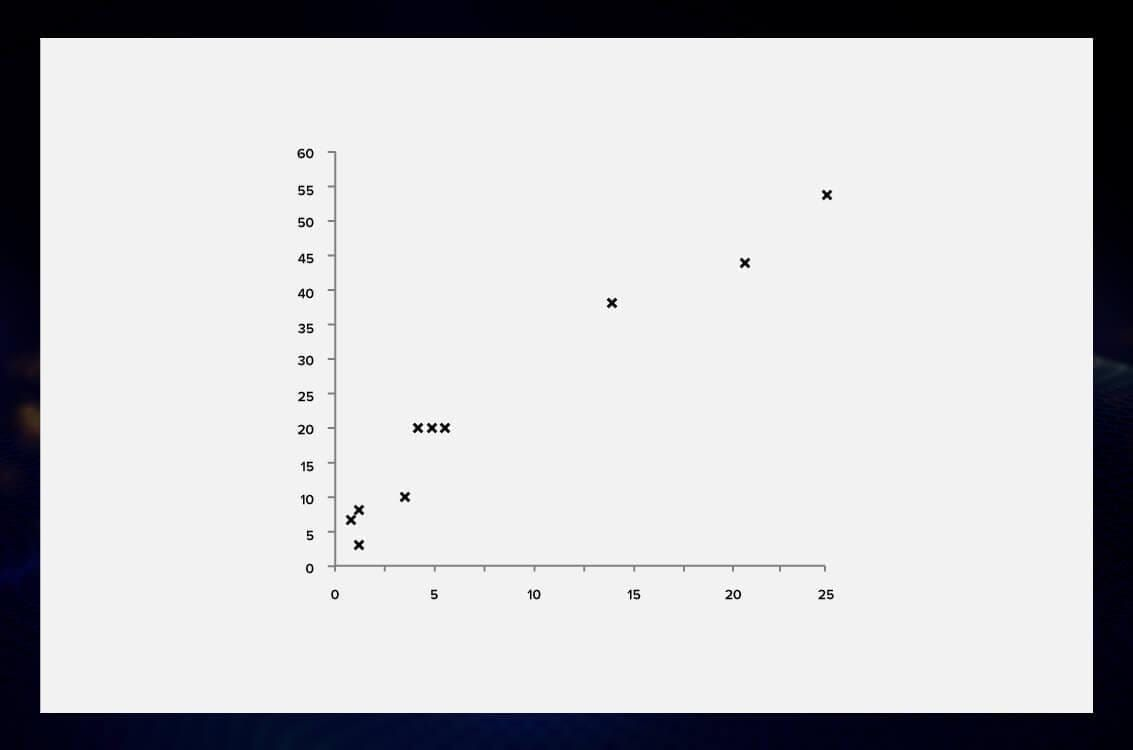We can see that there is a clear correlation between the marketing budget and the number of sold items.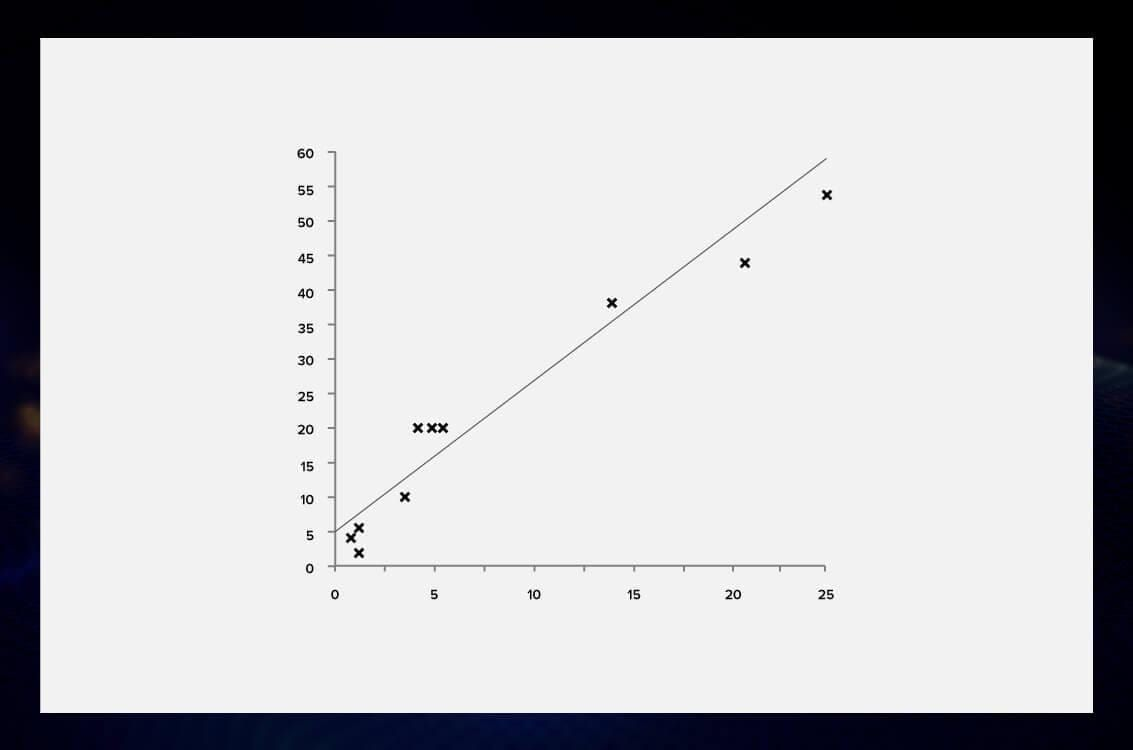Indeed, when we calculate the coefficient (again, using Acula.com), we get 0.967. The closer it is to one, the higher the correlation between the variables is. In this case, we see a strong positive correlation.

### 4. Check the relationship between the independent variables

An important step to build an accurate model is to check that there is no correlation between the independent variables. Otherwise, we won’t be able to tell which factor affects the output, and our efforts will be pointless.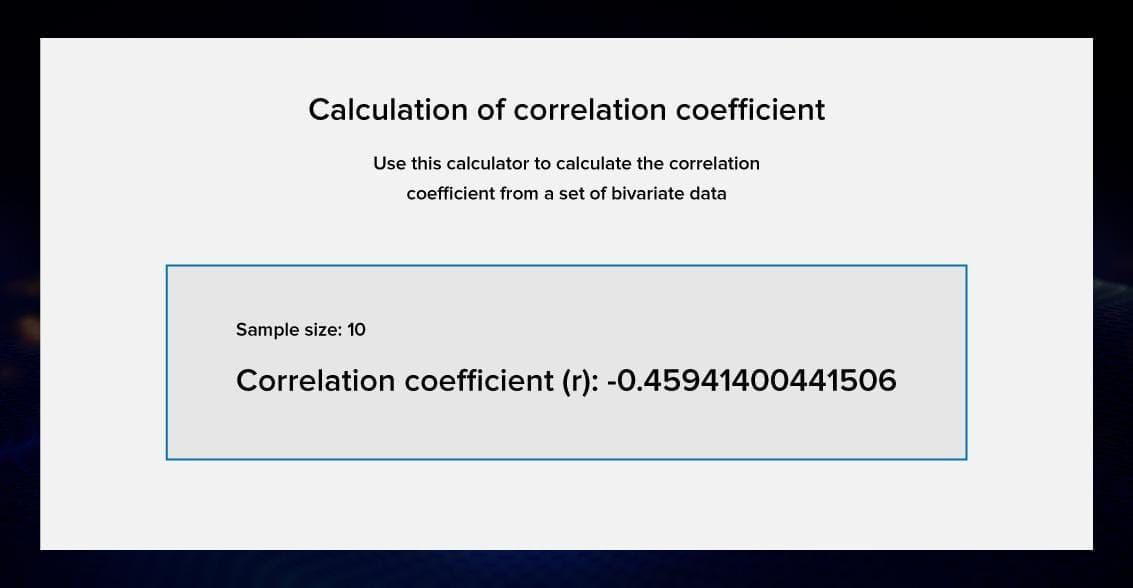Oh no, there is indeed a correlation between the two variables. What should we do?

### 5. Use non-redundant independent variables in analysis to discover the best fitting model

If you find yourself in a situation where there is a correlation between two independent variables, you are at risk. In specialized lingo, such variables are called redundant. If the redundancy is moderate, it can just affect the interpretation. However, they often add noise to your model. Some people believe that redundant variables are pure evil, and I cannot blame them.

So, in our case, we won’t use both of our variables for our predictions. Using our scatter plots, we can see a strong correlation between the marketing budget and the sales, so we will use the marketing budget variable for our model.

### 6. Use the ML model to make predictions

My example was largely simplified. In real life, you will probably have more than two variables to make predictions. You can use this plan to get rid of redundant or useless variables. Conduct these steps as many times as you need.

Now, you are ready to create a linear regression model using machine learning.

## Machine learning resources that mention regression models/algorithms